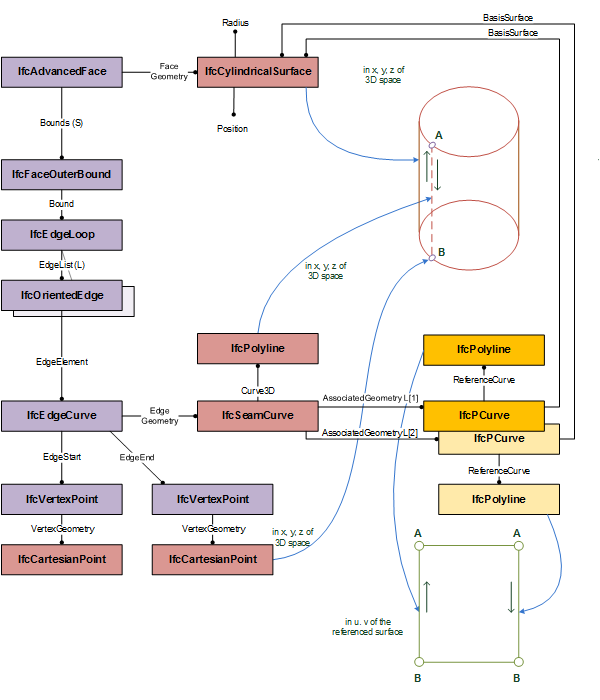# 8.9.3.60 IfcSeamCurve

## 8.9.3.60.1 Semantic definition

An IfcSeamCurve is a 3-dimensional curve that has additional representations provided by exactly two distinct pcurves describing the same curve at the two extreme ends of a closed parametric surface.

Figure 8.9.3.60.A shows an instance diagram explaining the use of IfcSeamCurve as a boundary curve of an IfcCylindricalSurface.Figure 8.9.3.60.A — use of a seam curve bounding a cylindrical surface

## 8.9.3.60.5 Formal representation

ENTITY IfcSeamCurve
SUBTYPE OF (IfcSurfaceCurve);
WHERE
SameSurface : IfcAssociatedSurface(SELF\IfcSurfaceCurve.AssociatedGeometry) = IfcAssociatedSurface(SELF\IfcSurfaceCurve.AssociatedGeometry);
TwoPCurves : SIZEOF(SELF\IfcSurfaceCurve.AssociatedGeometry) = 2;
END_ENTITY;